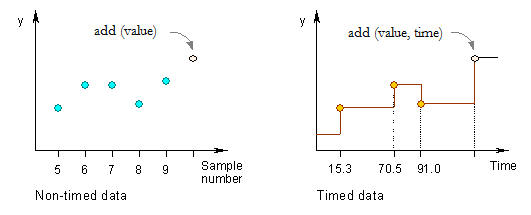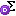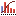AnyLogic

# Statistics

The Statistics object calculates statistical information (mean value, minimum, maximum, etc.) on a series of data samples of type double.

The object works differently depending on whether data are considered discrete or continuous.## Continuous data

Data samples are time-persistent; that is, value persists in continuous time but changes only at discrete time moments (like e.g. queue length). The samples must be added to this statistics with ascending time stamps. The mean, variance, etc. functions assume the last value added holds until the time of call. The mean of such dataset is a time-weighted value.

## Discrete data

Samples have no duration in time (like e.g. products cost or patients LOS). They occur as isolated, discrete points in time, so the mean is simply the sum of individual samples divided by number of samples.

To create a statistics object

1. Drag theStatistics element from theAnalysis palette into the graphical editor.
2. Go to the Properties view.
3. Specify, whether data samples should be handled as Discrete or Continuous.
4. Enter the expression evaluating the data item value in the Value edit box.
5. Finally, choose, how you want this data element to be updated.

## Properties

General

Name — The name of the statistics object. The name is used to identify and access the statistics.

Ignore — If selected, the statistics object is excluded from the model.

Visible — If selected, the statistics object is visible on a presentation at runtime.

Show name — If selected, the name of the statistics object is displayed on a presentation diagram.

Discrete (samples have no duration in time) — If selected, data are considered discrete.

Continuous (samples have duration in time) — If selected, data are considered continuous.

Value — Expression that will be dynamically evaluated to obtain the current data sample.

Update data automatically — If selected, new data samples are added automatically starting at the exact time (select the option Use model time) or date (select Use calendar dates) specified below and repeating with the specified Recurrence time.

Do not update data automatically — If selected, statistics is not updated automatically. In this case you should add new samples by yourself as described in Updating analysis data objects.

Log to database — If selected, data collected by this Statistics element will be added into the model execution log — statistics_log (if logging is turned on in the model's Database properties).

You can set the Statistics object to write data into the model execution log — statistics_log. To do this, select the statistics’ option Log to database, and enable the model to write to the log as described in Model execution logs.

While running the model, you can view the collected statistics and optionally copy it to the Clipboard so that later on you can paste it to some other application (e.g. to Excel) to perform statistical analysis.

## API for working with collected data

You can work with collected data using the API of the statistics element. A set of functions differs depending on the type of the Statistics element:

Continuous statistics
Function Description
void add(double value, double time) Adds a new data sample to the statistics, i.e. notifies the statistics about the value change at the given time.

value — the new value.
time — the time.
int count() Returns the number of samples added to the statistics.
void reset() Discards all statistics accumulated.
double max() Returns the maximum sample value, or -infinity if no samples have been added.
double min() Returns the minimum sample value, or infinity if no samples have been added.
String toString() Returns the tab-separated multiline textual representation of the statistics.
double deviation() Returns the deviation of the statistics at the time of last update.
double deviation(double time) Returns the standard deviation of the statistics at the given time assuming the last value added holds until the given time.
double integral() Returns the mean integral of the statistics at the time of last update, or 0 if no samples have been added.
double integral(double time) Returns the integral of the statistics at the given time assuming the last value added holds until the given time, or 0 if no samples have been added.
double mean() Returns the mean of the statistics at the time of last update, or 0 if no samples have been added.
double mean(double time) Returns the mean of the statistics at the given time assuming the last value added holds until the given time, or 0 if no samples have been added.
double meanConfidence() Returns the half-width mean confidence of the statistics at the time of last update, or 0 if no samples have been added. Mean confidence interval is calculated in assumption that confidence level is equal to 95%.
double meanConfidence(double time) Returns the half-width mean confidence interval of the statistics at the given time assuming the last value added holds until the given time, or infinity if less than 2 samples have been added or if no time has elapsed. Mean confidence interval is calculated in assumption that confidence level is equal to 95%.
double variance() Returns the variance of the statistics at the time of last update, or 0 if no samples have been added.
double variance(double time) Returns the variance of the statistics at the given time assuming the last value added holds until the given time, or 0 if no samples have been added.
Discrete statistics
Function Description